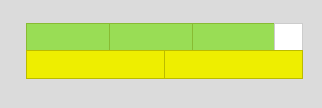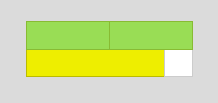#### You may also like### N000ughty Thoughts

How many noughts are at the end of these giant numbers?### DOTS Division

Take any pair of two digit numbers x=ab and y=cd where, without loss of generality, ab > cd . Form two 4 digit numbers r=abcd and s=cdab and calculate: {r^2 - s^2} /{x^2 - y^2}.### Mod 3

Prove that if a^2+b^2 is a multiple of 3 then both a and b are multiples of 3.

# Different by One

### Why do this problem?

This problem presents a context in which students can work systematically, notice surprising patterns, and make generalisations that they can explain visually, numerically or algebraically.

### Possible approach

Students could use Cuisenaire rods to work on this challenge, or the online Cuisenaire environment, or squared paper if rods are not available. Introduce the problem: invite students to choose two different colours of rod and make a line of each colour so that the lengths differ by the length of one white rod. You might like to show them the examples using yellow and green:Give students some time to explore, and then challenge students to look for general patterns in their results. One very fruitful line of enquiry, which leads to generalisations that might involve triangular numbers, is suggested in the problem:
• If you start with two rods that differ in length by 2, when is it possible to make two lines that differ by 1?
• How many of each rod will you need?
By working systematically, students can begin to make predictions about how many of each rod they would need, and how long the two lines would be, if they could use rods longer than 10 - for example, 23 and 25.

Some students might use algebra to justify the patterns they notice.

Another line of enquiry might be to consider how to generate other arrangements from a particular example.
For example:
2 greens is longer by 1 than 1 yellow
7 greens is longer by 1 than 4 yellows
12 greens is longer by 1 than 7 yellows

### Key questions

Using red (2) and pink (4), you can never make a difference of 1 - why not?
How can you tell whether it will be possible to make a difference of 1 for a particular pair of rods?
If you record your results in a systematic way, what patterns can you see? Can you prove that they will continue?

### Possible support

Encourage students to start by investigating
pink (4) and dark green (6)
yellow (5) and black (7)
dark green (6) and brown (8)
black (7) and blue (9)
For each pair, they could work systematically until they either find a combination that has a difference of one or have a convincing argument why it is impossible.

### Possible extension

Students could explore pairs of rods that can be arranged into lines whose lengths differ by two, three, four...